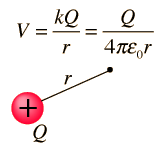Point Charge Potential

The electric potential (voltage) at any point in space produced by a point charge Q is given by the expression below. It is the electric potential energy per unit charge and as such is a characteristic of the electric influence at that point in space. Since it is a scalar quantity, the potential from multiple point charges is just the sum of the point charge potentials of the individual charges and can be extended to calculate the potential from a continuous charge distribution.Show

k = Coulomb's constant
At a distance r = x10^ m
from a charge Q = x10^ C
the voltage is V = x10^ volts.

Enter data, then click on the quantity you wish to calculate in the active formula above. Unspecified parameters will default to 1, but all values may be changed.

 Potentials for other charge geometries
Index

Voltage concepts

 HyperPhysics***** Electricity and Magnetism R Nave
Go Back

Point Charge Potential

The potential (voltage) of a point charge can be evaluated by calculating the work necessary to bring a test charge q in from an infinite distance to some distance r. The zero of potential is chosen at infinity.Considering a radial path from a to b, the work done by the Coulomb force is obtained from a line integral which in this case becomes just a polynomial integral since we are moving along a straight radial line:The change in potential is the work done per unit charge against the Coulomb force, soFor a positive charge q, the Coulomb force does positive work in moving it from a to b, so that represents a drop in potential energy.

Index

Voltage concepts

 HyperPhysics***** Electricity and Magnetism R Nave
Go Back